# Never Forget Anything Again with DropletMost of us that set reminders on our phones do so through a designated app or alarms. They go off at the same time each day or week and we simply swipe right to clear it from our phone’s screen. And, more often than not, we then forget to complete the task. The garbage is left indoors, birth control isn’t taken, and we even forget to hand in assignments. Droplet is here to make sure you don’t miss a beat or a pill.

Droplet is a tiny device that packs a large punch with reminders. It can attach to just about anything and, by syncing with your smartphone or tablet, will remind you to complete those mundane tasks that we so often forget to do. Each Droplet can be assigned a different task so, essentially, you could have a reminder for everything you need to do in life.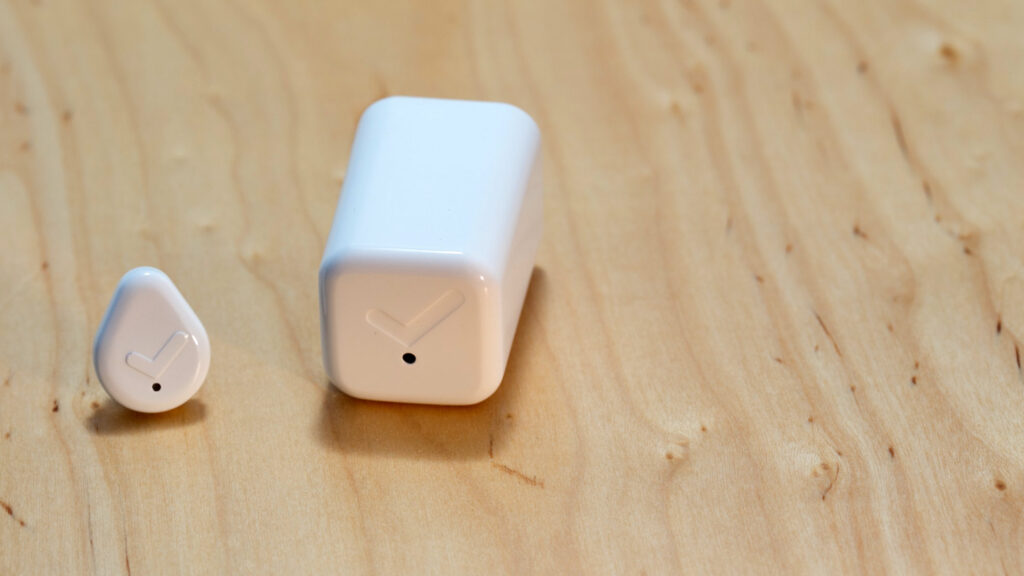It works by either connecting to your phone and the accompanying app via Bluetooth or through a smart hub (which can handle as many droplets as you’d like). First, you set up a task for each Droplet. Your task can be something like brushing for the full two minutes (who has the time?!) or taking out the bin. You can assign a time or a day of the week and even a person to remind for each task. The idea is that each time you take out the bin you’ll press Droplet which will mark the task as completed. When, and only when, you forget a task, the Droplet app will send you a reminder.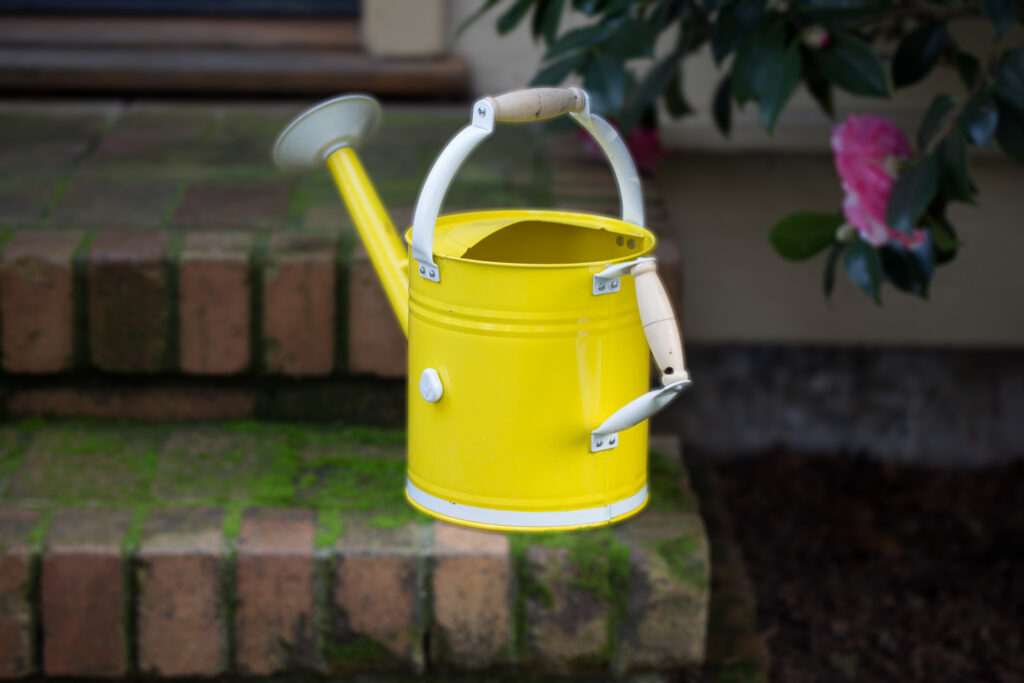You’ll need to press Droplet in order to mark the task as complete so you’re unable to just swipe right to clear the reminder. With that sort of accountability, it would be crazy to press Droplet without actually doing the task. This is the psychology that the device relies on to get your tasks done. As with other life tracking gadgets, the psychology behind goal setting and completion is the key to setting and sticking to new routines. At first, you’ll have small goals, such as a certain number of reps on the bench press. But, by staying consistent and having a gadget to answer to, you’ll soon do each task as part of your routine.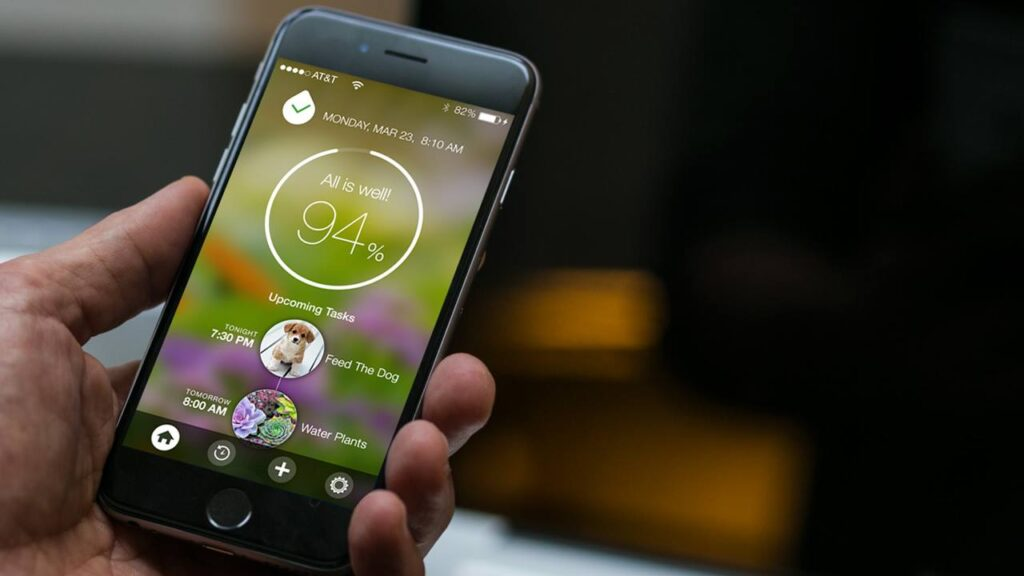The app is designed to get you into routines and to simplify oft-forgotten chores. You can set reminders to be once or twice or constant and nagging – whatever you need to get the task done. In addition, you can have the reminders set as phone calls or emails and even specific family members or roommates. With the app, you can set and reach your goals, track your progress to reveal trends, and, with a flexible platform along with IFTTT configurations, set tasks to be absolutely anything. You can even have it send an automatic “On my way home” text when you leave the office; you’ll just need to press it as you turn off the lights.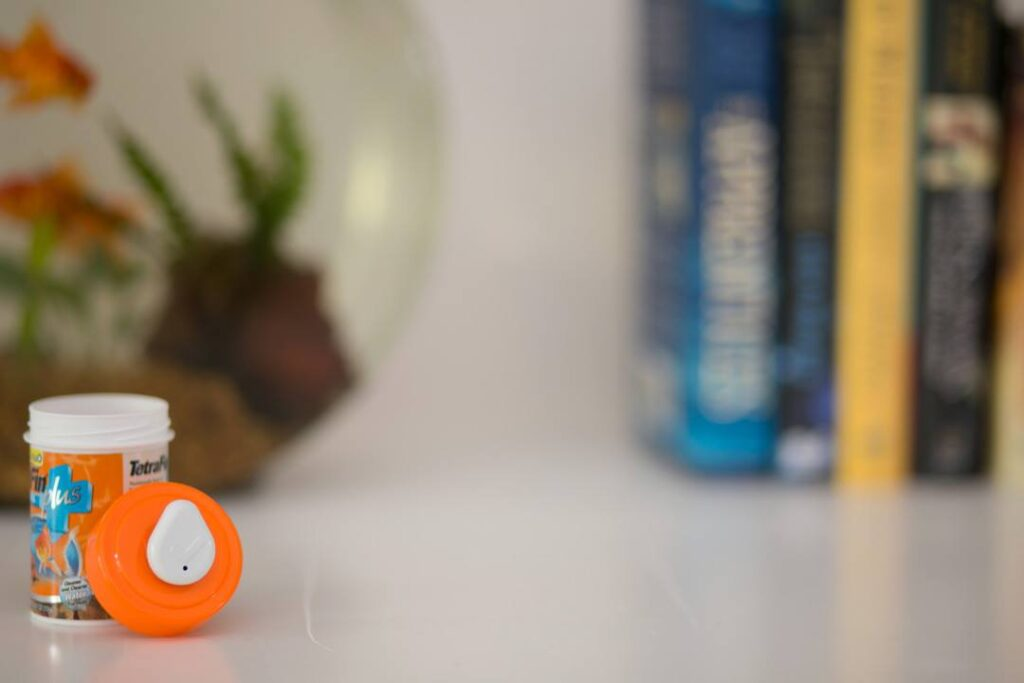With such a smart gadget that may need to be out in the open it will need to be small and inconspicuous. Coming in a glossy white finish, Droplet is just 26mm tall and 21mm wide and 7mm thick. Its adhesive backing can be stuck and re-stuck so you can switch the tasks and locations as you’d like. Droplet is splash-proof for now, but the designers are looking into extending that to waterproof with the increasing donations. Equipped with Bluetooth, it has a range of 100 feet. The smart hub is equally discreet at just 34x34mm. It has Bluetooth and Wi-Fi and only works with US sockets (unless you get an adapter).In the future, the Droplet team are looking to sort out a battery replacement program. At the moment, the battery in Droplet isn’t replaceable; you’re expected to replace the actual device. With the battery lasting over a year and at such a low price, it doesn’t seem like too much effort to do. However, for a gadget that should simplify our lives, it would just be another task on the list to be forgotten.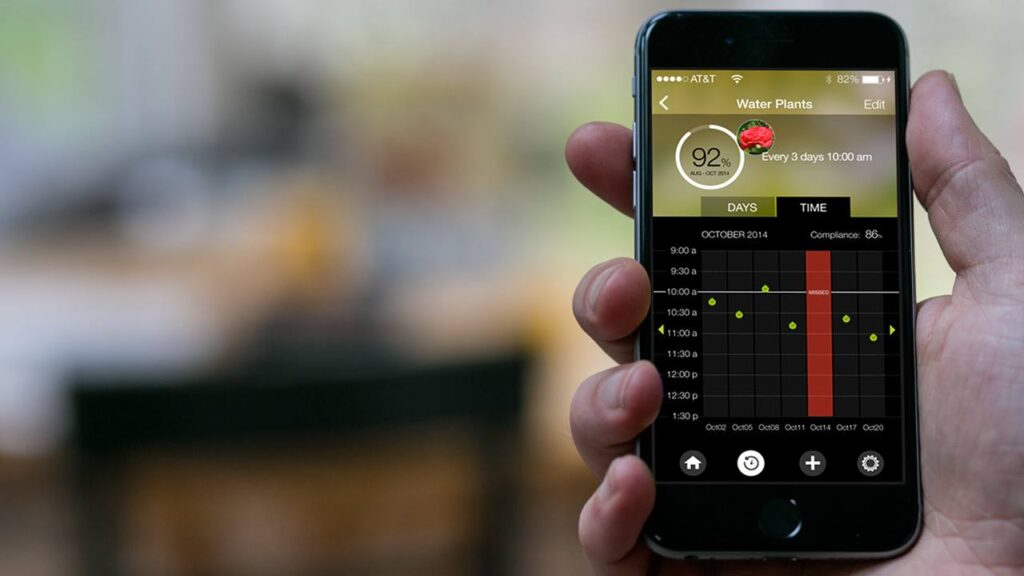Droplet is currently on track to fulfilling their \$80k goal on Kickstarter, which ends on May 19th. For just a \$19 (£12) pledge you can get a single Droplet and for \$39 (£25) you can get Droplet along with the smart hub. There are multipack options with different colours, which is great for the kids. The Droplet team expect to refine the product over the next few months and begin shipping in December 2015.

#### Meet Ashley Timms

Ashley Timms is the Editor in Chief at Gadget Flow where she gets to write about the most incredible products in the world. When she’s not writing, she’s producing fine art dog photography.

Click or drag your image here (Maximum Size 4MB, Accepted formats JPG, PNG, GIF).
• 😂
• ❤️
• ♥️
• 😍
• 😭
• 😊
• 😒
• 😘
• 💕
• ☺️
• 😩
• 👌
• 😔
• 😏
• 😁
• 😉
• 👍
• 🙏
• 😌
• 🎶
• 😳
• 🙌
• 🙈
• 😢
• 😎
• ✌️
• 👀
• 😅
• 😴
• 😄
• 💜
• 💔
• 💯
• 😑
• 💖
• 💙
• 😕
• 💁
• 😜
• 😞
• 😋
• 😐
• 😪
• 👏
• 💘
• 💗
• 💞
• ⬅️
• 🙊
• 💋
• 👉
• 🌸
• 😱
• 🔥
• 😡
• 😃
• 🎉
• 👊
• 😫
• 📷
• 🌹
• 😝
• 💪
• 💀
• ☀️
• 💛
• 😤
• 🌚
• 😆
• 😓
• 👈
• ✔️
• 😻
• 😀
• 😷
• 💚
• 👋
• 😣
• 💓
• ▶️
• ◀️
• ↪️
• ↩️
• 👑
• 😚
• 😛
• 😥
• 😇
• 🎧
• 😖
• 😠
• 😬
• 🌟
• 🔫
• 🙋
• 👎
• 💃
• 🎵
• 😶
• 💫
• 👇
• 🔴
• 🙅
• 💥
• 💭
• 👅
• 💩
• 😰
• 💎
• 🙆
• 🍕
• 😹
• 🌞
• 🍃
• 💦
• 🐧
• 💤
• 🚶
• ✈️
• 🎈
• 🎀
• ☑️
• 😟
• 🔞
• 😨
• 🍀
• 🌺
• 🎤
• 👐
• 👻
• 🌴
• ‼️
• 💅
• 👽
• 🙇
• 👼
• 👯
• ❄️
• ☝️
• 😙
• 🌈
• 🌙
• 💟
• 💝
• 🎁
• 🍻
• 😧
• 🌍
• 🎥
• ♣️
• ✖️
• 🏃
• 🌻
• 🌎
• 💐
• 🐶
• 💰
• 🌿
• 👫
• 🍂
• 🌷
• 🎂
• 🐱
• 😵
• 👆
• 😮
• 😯
• 🏀
• 🎄
• 💍
• 🌝
• 😲
• 👭
• 💸
• 😿
• 🙉
• 💨
• 🌵
• ♨️
• ☎️
• 🍁
• 👸
• 💆
• 💌
• 🏆
• 🙍
• 🎊
• 🌼
• 🔪
• 👄
• 🍟
• 🍩
• 😦
• 🌊
• 💣
• 🆗
• 🌀
• 🚀
• 💏
• 🍭
• 🎬
• 🐷
• 😈
• 👿
• 🐝
• 😽
• 💢
• 🎼
• 🎅
• 🌏
• 🏈
• 🎸
• ♦️
• 🐼
• 💬
• 🍓
• 😼
• 🍌
• 🍉
• 😸
• ♠️
• 🔝
• 🍆
• 🔮
• 🍴
• 📲
• 📱
• ⚠️
• 🙀
• 🔸
• 👶
• 🐾
• 👣
• 🍺
• 🍷
• 📹
• 🐰
• 🍹
• 🚬
• 👾
• 🍑
• 🐍
• 🐢
• 🍒
• 😗
• 🐸
• 🌌
• 🚨
• 🐣
• 📕
• 🍬
• 🍔
• 🐻
• 🐯
• 🚗
• 🍦
• 🍍
• 🌾
• 💉
• 🚮
• 🍫
• ▪️
• 📺
• 💊
• 🐙
• 🎃
• 🍇
• 😺
• 💿
• 🍸
• 🍰
• 🎮
• ™️
• ⬇️
• 🚫
• 💄
• 🐳
• 📝
• ®️
• 🍪
• 🐬
• 🔊
• 👨
• 🐥
• 🐒
• 📚
• 👹
• 💂
• 📢
• ✂️
• 👧
• 🎓
• ⚾️
• 🚦
• 👩
• 🎆
• 🌠
• 🆘
• 🍄
• 😾
• 🛅
• 👠
• 🎯
• 🏊
• 🔑
• 👙
• 👪
• 🐘
• 💧
• 🌱
• 🍎
• 🆒
• 📞
• 💵
• 🏡
• 📖
• 💇
• 💻
• 💡
• 🔙
• 👦
• 🔐
• 🙎
• 🍊
• ↔️
• 🌅
• 🍗
• 🔵
• 🚘
• 🍧
• 🐦
• 🌛
• 👓
• 🐐
• 🌃
• 👵
• 🌑
• 👬
• 🛃
• 🐠
• 🏠
• 🔃
• 🌜
• 📍
• 🌕
• 👟
• 🍋
• 🍼
• 🎨
• ✉️
• 🍝
• 🎐
• 🍥
• 🌲
• 🆙
• ⬆️
• ↗️
• ↘️
• ↙️
• 🎭
• 👃
• 🐽
• 🐟
• 👳
• 🐨
• 👂
• ✳️
• 🔹
• 🚿
• 🐛
• 🍜
• 🎩
• 👰
• 🏁
• 🐴
• 🐵
• 🚼
• 🆕
• 🆓
• 🎇
• 🌽
• 🎾
• 🔋
• 🐺
• 🗿
• 🐮
• 📣
• 👴
• 👗
• 🔗
• 🐔
• 🍳
• 🐋
• 🌳
• 🍱
• 📌
• 🔜
• 🔁
• 🐉
• 🐹
• 🏄
• 🐭
• 🌒
• 🚙
• 🅰️
• ⁉️
• 🔌
• 🌓
• 🔱
• 🍞
• 👮
• 🍵
• 🎣
• 🌔
• 🚲
• 👤
• 🍚
• 📻
• 🐤
• ⤵️
• 🌘
• 🌗
• 🔘
• 🐑
• 👱
• 🌖
• 🔒
• 🍏
• 👺
• 🚩
• 🔄
• 🐎
• 🍤
• 🌄
• 🌋
• 🐓
• 📥
• 💒
• 🍣
• 🍨
• 🍅
• 🐇
• ✴️
• 🔺
• 🔆
• 👲
• 🏪
• 👥
• 🐞
• 🔻
• ⤴️
• 📛
• 🛀
• 🐊
• 🌰
• 🐕
• 🐈
• 🔨
• 🍖
• 🐚
• ❇️
• 🅱️
• Ⓜ️
• 🐩
• 🍲
• 👖
• 🍯
• 🎹
• 🔓
• 🗽
• 💲
• 🏂
• 💮
• 👔
• 💠
• 🚺
• 🐜
• 🌇
• 🅾️
• 🐲
• 🐌
• 📀
• 👕
• 🎲
• 🎎
• 🎱
• 🚌
• 🍮
• 🎌
• 〽️
• 🐫
• 🍛
• 🚂
• 🏥
• 🔷
• 🎋
• 🔔
• 🍐
• 🔶
• 🌐
• 🚪
• 🕕
• 🚔
• 📩
• 🌂
• 🎷
• 🚴
• 🍡
• 🏢
• 🚣
• 👒
• 👞
• 🏩
• 🗻
• 🐪
• 👜
• 🎺
• 🏫
• 🐄
• 🌆
• 👷
• 🚽
• 🐖
• 🔰
• 🎻
• 🔛
• 💳
• 🆔
• 🎡
• 🎳
• 💈
• 👛
• 🎢
• 🐀
• 📅
• 🏉
• 🐏
• 🔼
• 🔲
• 📴
• 🗼
• 👘
• 🚢
• 🔎
• 🔍
• 🚒
• 🕦
• 🚓
• 🃏
• 🌉
• 📦
• 🚖
• 📆
• 🏇
• 🐅
• 👢
• 🚑
• 🔳
• 🐗
• 🎒
• 💷
• 🐂
• 🍙
• 🆚
• 🔚
• 🅿️
• 👡
• 💺
• 🚕
• 💼
• 📰
• 🎪
• 🔯
• 🚹
• 🏰
• 🔦
• 🌁
• 🎍
• 🎫
• 🚁
• 💽
• 🚍
• 🍈
• 🏤
• 🔟
• 📓
• 🔕
• 🍢
• 🎏
• 🎠
• 🐡
• 📈
• 🍠
• 🎿
• 🕛
• 📶
• 🚧
• #
• 📡
• 💶
• 👚
• 📒
• 🐆
• 🔅
• 🕒
• 🏬
• 🚚
• 🍶
• 🚃
• 🚤
• 📼
• 🕐
• 🐃
• 🔽
• 💴
• 🔇
• 🎽
• 🕑
• 📎
• 🏧
• 🎦
• 🔭
• 🎑
• 📘
• ◻️
• 📮
• 📧
• 🐁
• 🚄
• 🔩
• 🆖
• 🏨
• 🚾
• 🏮
• 🔂
• 📬
• 📉
• 📗
• 🚜
• 🚇
• 📋
• 📵
• 🕓
• 🚭
• 🎰
• 🕔
• 🛁
• 📜
• 🚉
• 🍘
• 🏦
• 🔧
• 🈯️
• 🚛
• 📄
• 📊
• 🚷
• 📳
• 🕙
• 🕘
• 🚅
• 🚐
• 🚊
• 🕗
• 🈳
• 🚥
• 🚵
• 🔬
• 🏯
• 🔖
• 📑
• 👝
• 🆎
• 📃
• 🎴
• 🕚
• 📠
• 🕖
• 💱
• 🔉
• 💹
• 🆑
• 💾
• 🏣
• 🔈
• 🗾
• 🈺
• 🀄
• 📨
• 📙
• 🚻
• 🈚️
• 🈶
• 📐
• 🚋
• 🈸
• 🚎
• 🈷
• 🔢
• 📔
• 🈲
• 🈵
• 📯
• 🏭
• 🚸
• 🚆
• 📏
• 📟
• 🉑
• 🈴
• 🔏
• 🕜
• 🈂️
• 📤
• 🔀
• 📫
• 🚈
• 🕤
• 🚏
• 📂
• 📁
• 🚰
• 📇
• 🕝
• 🚝
• 🕧
• 🕥
• 🔤
• 📪
• 🕟
• 🚞
• 🚯
• 🕞
• 🕢
• 🕠
• 🔠
• 📭
• 🔣
• 🚡
• 🕣
• 🕡
• 🔡
• 🚠
• 🈁
• 🛂
• 🚱
• 🚟
• 🛄
• 🚳
• 🏳‍🌈
• 🕵
• 🤗
• 🤖
• 🤕
• 🤔
• 🤓
• 🤒
• 🤑
• 🤐
• 🙄
• 🙃
• 🙂
• 🙁
• 🤘
• 🖖
• 🖕
• 🖐
• 🕶
• 👁‍🗨
• 👁
• 🏋
• 🕴
• 🏌
• ❣️
• 🛢
• 🛡
• 🛠
• 🛏
• 🛎
• 🛍
• 🛌
• 🛋
• 🗳
• 🗡
• 🗞
• 🗝
• 🗜
• 🗓
• 🗒
• 🗑
• 🗄
• 🗃
• 🗂
• 🖼
• 🖲
• 🖱
• 🖨
• 🖥
• 🖍
• 🖌
• 🖋
• 🖊
• 🖇
• 🕹
• 🕳
• 🕰
• 🕯
• 📿
• 📽
• 📸
• 🏺
• 🏷
• 🏴
• 🏳
• 🎞
• 🎛
• 🎚
• 🎙
• 🌡
• 🛳
• 🛰
• 🛬
• 🛫
• 🛩
• 🛥
• 🛤
• 🛣
• 🗺
• 🕍
• 🕌
• 🕋
• 🏟
• 🏞
• 🏝
• 🏜
• 🏛
• 🏚
• 🏙
• 🏘
• 🏗
• 🏖
• 🏕
• 🏔
• 🏎
• 🏍
• 🏹
• 🏸
• 🏵
• 🏓
• 🏒
• 🏑
• 🏐
• 🏏
• 🏅
• 🎟
• 🎗
• 🎖
• 🧀
• 🍿
• 🍾
• 🍽
• 🌶
• 🌯
• 🌮
• 🌭
• ☃️
• ☂️
• 🦄
• 🦃
• 🦂
• 🦁
• 🦀
• 🕸
• 🕷
• 🕊
• 🐿
• 🌬
• 🌫
• 🌪
• 🌩
• 🌨
• 🌧
• 🌦
• 🌥
• 🌤
• 🗣
• 🖤
• 🗨
• 🥚
• 🛑
• 🥁